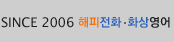Home > 강사소개 > 강사수업샘플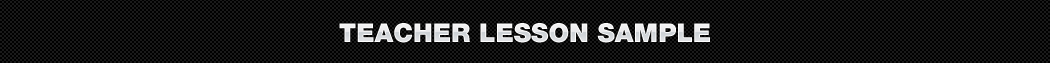Teacher Chloe's Sample Class- romelyn1님
작성일 : 2023-02-23 00:06:25   조회수 : 33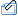audio1827458434.mp3 (19.06 MB)

PRONUNCIATION:

1. fan = [ fahn ]

2. beside = [ bih-sahyd ]

3. can = [ Kuhn ]

4. bat = [ bat ]

5. leg = [ leg ]

6. jet = [ jet ]

7. color = [ kuhl-er ]

GRAMMAR:

1. Yes = Yes, I am happy.

2. Pork = I ate pork for dinner.

3. Yes = Yes, it was yummy.

4. One = The answer is number one.

5. Sentence two = The answer is in sentence number two.

6. Looking at the hat = The cat is looking at the hat.

7. Mat = The mat is under the cat.

8. Fan = The fan is beside the cat.

9. Red = It is red.

10. Brown = It is brown.

11. Two leg = I have two legs.

12. White = It is white.

13. No = No, I don't.

14. Blue = My favorite color is blue.

15. Yes = Yes, I do.

16. Two men = There are two men in the picture.

HOMEWORK: ​ If you could bring an animal to school what would it be and why?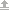최신목록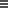검색목록
 의견나누기 | 의견을 올려주세요.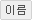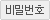등록권한이 없습니다.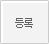현재 0 / 최대 2000 byte - 한글 1000자, 영문 2000자)